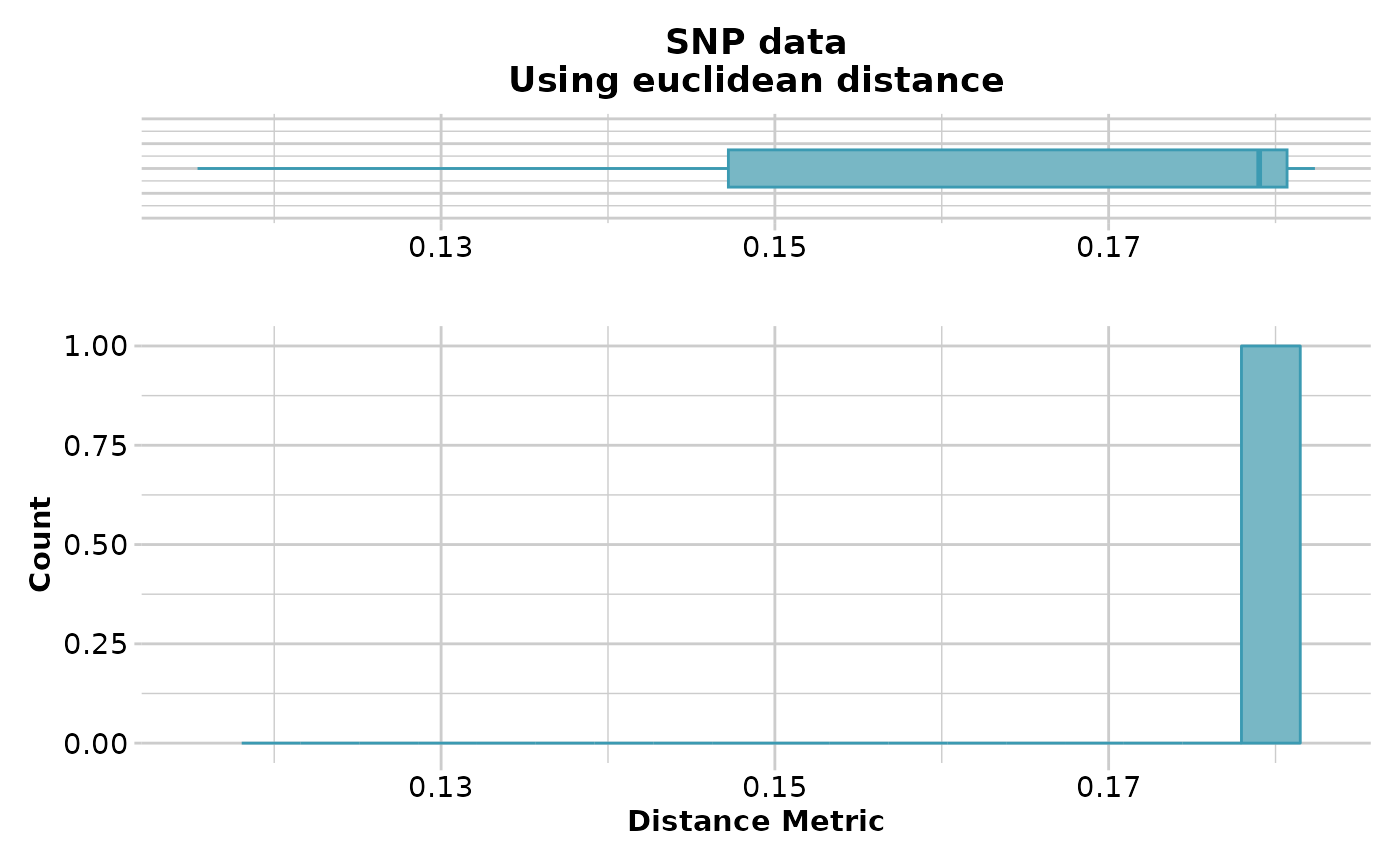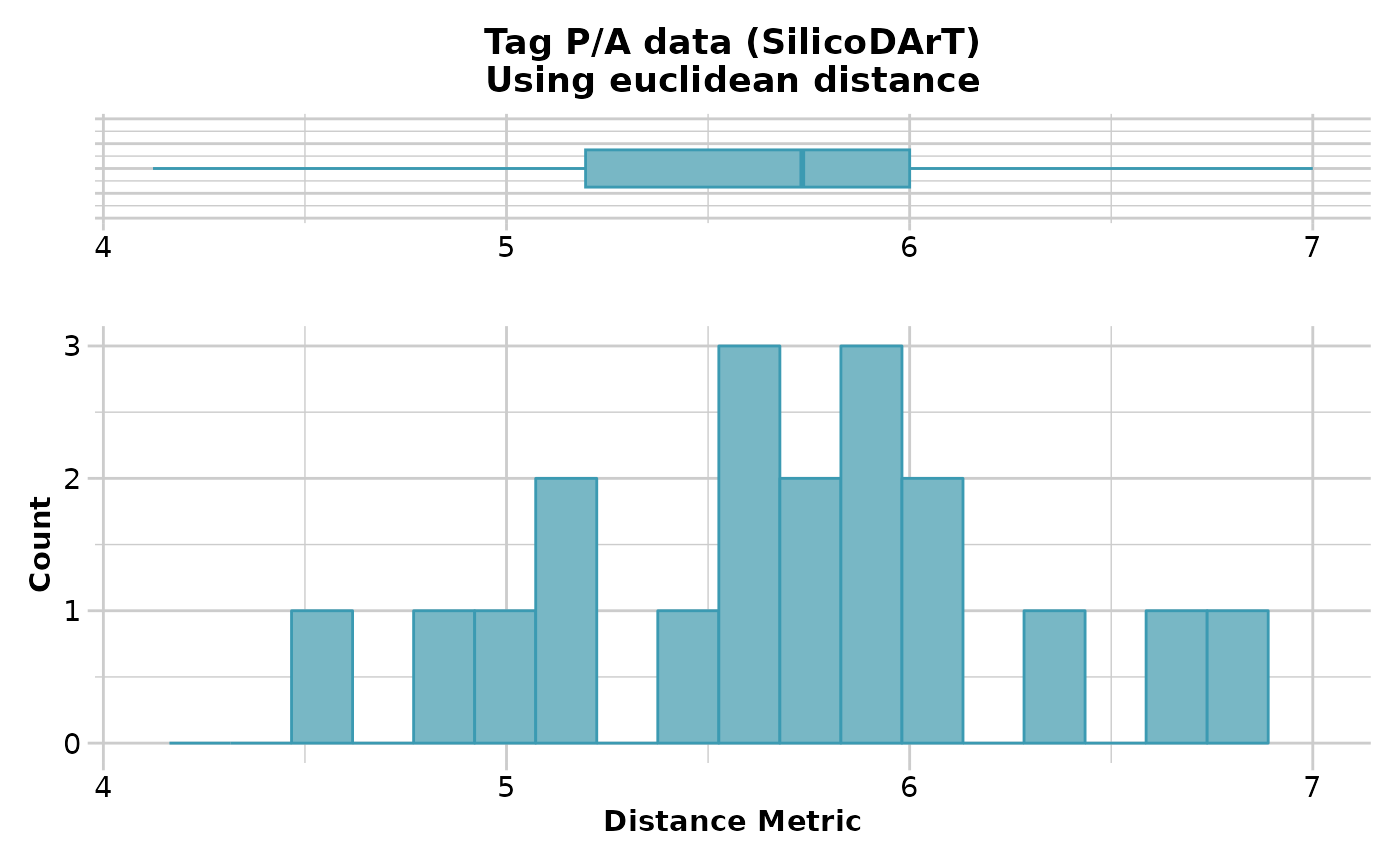This script calculates various distances between populations based on allele frequencies (SNP genotypes) or frequency of presences in presence-absence data (Euclidean and Fixed-diff distances only).

## Usage

gl.dist.pop(
x,
method = "euclidean",
plot.out = TRUE,
scale = FALSE,
output = "dist",
plot_theme = theme_dartR(),
plot_colors = two_colors,
save2tmp = FALSE,
verbose = NULL
)

## Arguments

x

Name of the genlight containing the SNP genotypes [required].

method

Specify distance measure [default euclidean].

plot.out

If TRUE, display a histogram of the genetic distances, and a whisker plot [default TRUE].

scale

If TRUE and method='Euclidean', the distance will be scaled to fall in the range [0,1] [default FALSE].

output

Specify the format and class of the object to be returned, dist for a object of class dist, matrix for an object of class matrix [default "dist"].

plot_theme

User specified theme [default theme_dartR()].

plot_colors

Vector with two color names for the borders and fill [default two_colors].

save2tmp

If TRUE, saves any ggplots and listings to the session temporary directory (tempdir) [default FALSE].

verbose

Verbosity: 0, silent or fatal errors; 1, begin and end; 2, progress log ; 3, progress and results summary; 5, full report [default 2 or as specified using gl.set.verbosity].

## Value

An object of class 'dist' giving distances between populations

## Details

The distance measure can be one of 'euclidean', 'fixed-diff', 'reynolds', 'nei' and 'chord'. Refer to the documentation of functions described in the the dartR Distance Analysis tutorial for algorithms and definitions.

## Author

author(s): Arthur Georges. Custodian: Arthur Georges -- Post to https://groups.google.com/d/forum/dartr

## Examples

 # \donttest{
# SNP genotypes
D <- gl.dist.pop(possums.gl[1:90,1:100], method='euclidean')
#> Starting gl.dist.pop
#>   Processing genlight object with SNP data
#>   Calculating distances:  euclidean
#>   Refer to the dartR Distance Analysis tutorial for algorithms
#>#>   Returning a stats::dist object
#> Completed: gl.dist.pop
#>
D <- gl.dist.pop(possums.gl[1:90,1:100], method='euclidean',scale=TRUE)
#> Starting gl.dist.pop
#>   Processing genlight object with SNP data
#>   Calculating distances:  euclidean
#>   Refer to the dartR Distance Analysis tutorial for algorithms
#>#>   Returning a stats::dist object
#> Completed: gl.dist.pop
#>
if (FALSE) {
#D <- gl.dist.pop(possums.gl, method='nei')
#D <- gl.dist.pop(possums.gl, method='reynolds')
#D <- gl.dist.pop(possums.gl, method='chord')
#D <- gl.dist.pop(possums.gl, method='fixed-diff')
}
#Presence-Absence data [only 10 individuals due to speed]
D <- gl.dist.pop(testset.gs[1:10,], method='euclidean')
#> Starting gl.dist.pop
#>   Processing genlight object with Presence/Absence (SilicoDArT) data
#>   Calculating distances:  euclidean
#>   Refer to the dartR Distance Analysis tutorial for algorithms
#>#>   Returning a stats::dist object
#> Completed: gl.dist.pop
#>
# }
res <- gl.dist.pop(platypus.gl)
#> Starting gl.dist.pop
#>   Processing genlight object with SNP data
#>   Warning: data include loci that are scored NA across all individuals.
#>   Consider filtering using gl <- gl.filter.allna(gl)
#>   Calculating distances:  euclidean
#>   Refer to the dartR Distance Analysis tutorial for algorithms
#>#>   Returning a stats::dist object
#> Completed: gl.dist.pop
#>